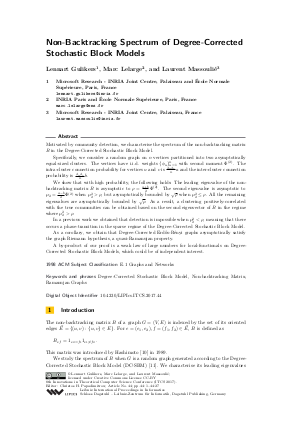Document# Non-Backtracking Spectrum of Degree-Corrected Stochastic Block Models

### Authors Lennart Gulikers, Marc Lelarge, Laurent Massoulié## File

LIPIcs.ITCS.2017.44.pdf
• Filesize: 0.54 MB
• 27 pages

## Cite As

Lennart Gulikers, Marc Lelarge, and Laurent Massoulié. Non-Backtracking Spectrum of Degree-Corrected Stochastic Block Models. In 8th Innovations in Theoretical Computer Science Conference (ITCS 2017). Leibniz International Proceedings in Informatics (LIPIcs), Volume 67, pp. 44:1-44:27, Schloss Dagstuhl - Leibniz-Zentrum für Informatik (2017)
https://doi.org/10.4230/LIPIcs.ITCS.2017.44

## Abstract

Motivated by community detection, we characterise the spectrum of the non-backtracking matrix B in the Degree-Corrected Stochastic Block Model. Specifically, we consider a random graph on n vertices partitioned into two asymptotically equal-sized clusters. The vertices have i.i.d. weights {\phi_u}_{u=1}^n with second moment \PHItwo. The intra-cluster connection probability for vertices u and v is \frac{\phi_u \phi_v}{n}a and the inter-cluster connection probability is \frac{\phi_u \phi_v}{n}b. We show that with high probability, the following holds: The leading eigenvalue of the non-backtracking matrix B is asymptotic to \rho = \frac{a+b}{2} \PHItwo. The second eigenvalue is asymptotic to \mu_2 = \frac{a-b}{2} \PHItwo when \mu_2^2 > \rho, but asymptotically bounded by \sqrt{\rho} when \mu_2^2 \leq \rho. All the remaining eigenvalues are asymptotically bounded by \sqrt{\rho}. As a result, a clustering positively-correlated with the true communities can be obtained based on the second eigenvector of B in the regime where \mu_2^2 > \rho. In a previous work we obtained that detection is impossible when $\mu_2^2 \leq \rho,$ meaning that there occurs a phase-transition in the sparse regime of the Degree-Corrected Stochastic Block Model. As a corollary, we obtain that Degree-Corrected Erdös-Rényi graphs asymptotically satisfy the graph Riemann hypothesis, a quasi-Ramanujan property. A by-product of our proof is a weak law of large numbers for local-functionals on Degree-Corrected Stochastic Block Models, which could be of independent interest.
##### Keywords
• Degree-Corrected Stochastic Block Model
• Non-backtracking Matrix
• Machine Learning
• Social Networks

## Metrics

• Access Statistics
• Total Accesses (updated on a weekly basis)
0

## References

1. B. Bollobás, S. Janson, and O. Riordan. The phase transition in inhomogeneous random graphs. Random Struct. Algorithms, 31(1):3-122, August 2007.2. C. Bordenave, M. Lelarge, and L. Massoulié. Non-backtracking spectrum of random graphs: community detection and non-regular ramanujan graphs. arXiv preprint 1501.06087, 2015.3. A. Decelle, F. Krzakala, C. Moore, and L. Zdeborová. Asymptotic analysis of the stochastic block model for modular networks and its algorithmic applications. Phys. Rev. E, 84:066106, Dec 2011.4. W. Evans, C. Kenyon, Y. Peres, and L. Schulman. Broadcasting on trees and the ising model. Ann. Appl. Probab., 10(2):410-433, 05 2000.5. Z. Füredi and J. Komlós. The eigenvalues of random symmetric matrices. Combinatorica, 1(3):233-241, 1981.6. L. Gulikers, M. Lelarge, and L. Massoulié. An impossibility result for reconstruction in a degree-corrected planted-partition model. arXiv preprint 1511.00546, 2015.7. L. Gulikers, M. Lelarge, and L. Massoulié. A spectral method for community detection in moderately-sparse degree-corrected stochastic block models. arXiv preprint 1506.08621, 2015.8. P. Holland, K. Laskey, and S. Leinhardt. Stochastic blockmodels: First steps. Social Networks, 5(2):109-137, June 1983.9. M. Horton, H. Stark, and T. Terras. What are zeta functions of graphs and what are they good for? Contemporary Mathematics, Quantum Graphs and Their Applications;, 415:173-190, 2006.10. K. i. Hashimoto. Zeta functions of finite graphs and representations of p-adic groups. In Automorphic Forms and Geometry of Arithmetic Varieties, volume 15 of Advanced Studies in Pure Mathematics, pages 211 - 280. Academic Press, 1989.11. B. Karrer and M. Newman. Stochastic blockmodels and community structure in networks. Phys. Rev. E, 83:016107, Jan 2011.12. H. Kesten and B. P. Stigum. Additional limit theorems for indecomposable multidimensional galton-watson processes. Ann. Math. Statist., 37(6):1463-1481, 12 1966.13. H. Kesten and B. P. Stigum. A limit theorem for multidimensional galton-watson processes. Ann. Math. Statist., 37(5):1211-1223, 10 1966.14. F. Krzakala, C. Moore, E. Mossel, J. Neeman, A. Sly, L. Zdeborová, and P. Zhang. Spectral redemption in clustering sparse networks. Proceedings of the National Academy of Sciences, 110(52):20935-20940, 2013.15. A. Lubotzky, R. Phillips, and P. Sarnak. Ramanujan graphs. Combinatorica, 8(3):261-277, 1988.16. L. Massoulié. Community detection thresholds and the weak ramanujan property. ACM Symposium on the Theory of Computing (STOC), 2014.17. E. Mossel, J. Neeman, and A. Sly. A proof of the block model threshold conjecture. arXiv preprint 1311.4115, 2015.18. E. Mossel, J. Neeman, and A. Sly. Reconstruction and estimation in the planted partition model. Probability Theory and Related Fields, 162(3):431-461, 2015.19. R. Murty. Ramanujan graphs. J. Ramanujan Math. Soc., 18(1):1-20, 2003.X

Feedback for Dagstuhl Publishing